# Concave and Convex Mirrors – Ray Diagrams, Image Formation, Applications

• Difficulty Level : Easy
• Last Updated : 28 Mar, 2021

Light is responsible for anything around us visible. This happens when light rays are reflected to the observer from the source object. For a good reflection, the light source must be a polished or shiny surface that acts as a mirror. This is due to the phenomenon called reflection of light which does not intend to change the velocity of light it only reverses the direction of light incident on it. In order to obtain a good reflection of light, the surface chosen for the same must be spherical, smooth & shiny. Therefore, the mirror that is known as a spherical mirror is made out by cutting out a hollow spherical glass ball. A spherical mirror has a reflecting surface that can be inside or outside, it depends on the purpose of use.

## Spherical Mirrors

The curved surface we see of a shining spoon can be considered as a curved mirror. The most widely used type of curved mirror is the spherical mirror. The reflecting surface of such mirrors is considered to form a part of the surface of any sphere. Those mirrors which possess reflecting surfaces which are spherical are called spherical mirrors.

Attention reader! All those who say programming isn't for kids, just haven't met the right mentors yet. Join the  Demo Class for First Step to Coding Course, specifically designed for students of class 8 to 12.

The students will get to learn more about the world of programming in these free classes which will definitely help them in making a wise career choice in the future.

### Types of Spherical Mirrors

Spherical Mirrors are categorized into two types:

• Concave Mirrors
• Convex Mirrors

### Concave Mirror

If a hollow sphere is cut into some parts and the outer surface of the cut part is painted, then it turns out to be a mirror with its inner surface as the reflecting surface. This makes a concave mirror.

A concave mirror or converging mirror is a type of mirror that is bent towards the inwards side in the middle. Moreover, by looking in this mirror, we will feel that we are looking in a cave. We tend to use the mirror equation to deal with a concave mirror.

The equation for these mirrors determines position of the object and the accurate size of the object. The angle of incidence in the concave mirror is not same as the angle of reflection. Moreover, the angle of reflection in this case depends on the area on which the light hits.

Properties of Concave Mirrors:

• Light after reflection converges at a point when it strikes and reflects back from the reflecting surface of the concave mirror. Hence, it is also termed as converging mirror.
• When the converging mirror is placed very near to the object, a magnified and virtual image is observed.
• But, if we tend to increase the distance between the object and the mirror then the size of the image reduces and a real image is formed.
• The image formed by the concave mirror can be small or enlarged or can be either real or virtual.

Applications of Concave Mirrors:

• Used in shaving mirrors: Converging mirrors are most widely used in shaving because they have reflective and curved surfaces. At the time of shaving, the concave mirror forms an enlarged as well as an erect image of the face when the concave mirror is held closer to the face.
• Concave mirror used in the ophthalmoscope: These mirrors are used in optical instruments as in Ophthalmoscope for treatment.
• Uses of concave mirror in astronomical telescopes: These mirrors are also widely used in making astronomical telescopes. In an astronomical telescope, a converging mirror of a diameter of about 5 meters or more is used as the objective.
• Concave mirrors used in the headlights of vehicles: Converging mirrors are widely used in headlights of automobiles and in the motor vehicles, torchlights, railway engines, etc. as reflectors. The point light source is kept at the focus of the mirror, so after reflection, the light rays travel over a huge distance as parallel light beams of high intensity.
• Used in solar furnaces: Large converging mirrors are used to focus the sunlight to produce heat in the solar furnace. They are often used in solar ovens to gather a large amount of solar energy in the focus of the concave mirror for heating, cooking, melting metals, etc.

### Image Formation By Concave Mirror And Their Ray Diagrams

• When the object is kept at infinity:

As the parallel rays coming from the object converge at the principal focus, F of a concave mirror; after reflection through it. Therefore, when the object is at infinity the image will form at F.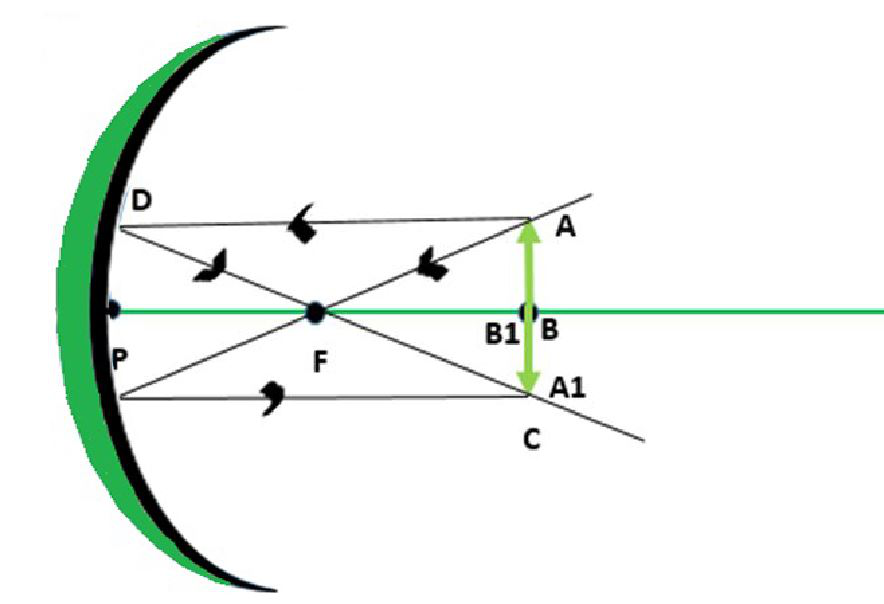Object at Infinity

Properties of the image formed: Point-sized image, highly diminished in size, Real and inverted image.

• When the object is placed between infinity and Centre of Curvature:

When the object is placed between infinity and the centre of curvature of a concave mirror then the image is formed between centre of curvature (C) and focus (F).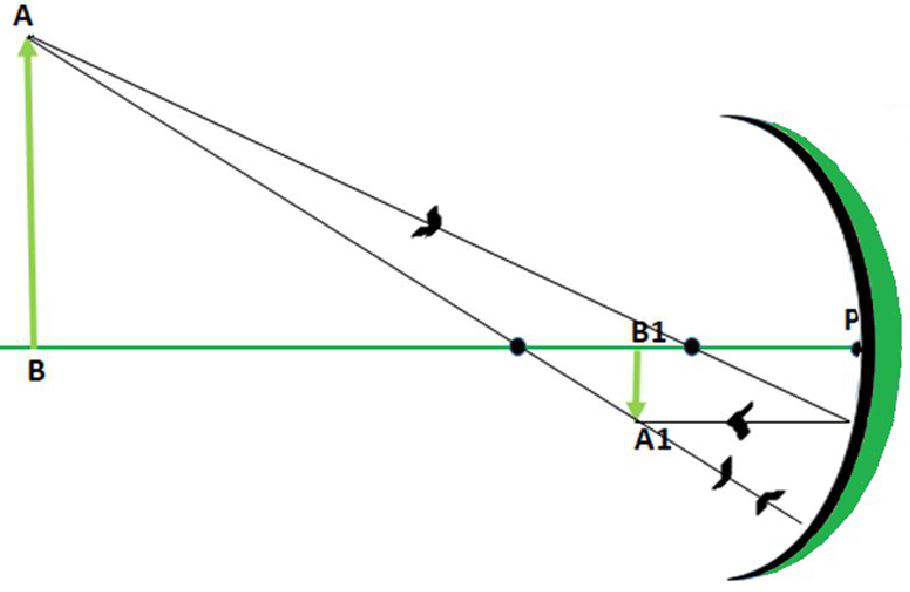Object between Infinity and C

Properties of image: It is diminished as compared to the object and alsoreal and inverted.

• Object at Centre of Curvature (C):

Whenever we place our object at the centre of curvature (C) of a concave mirror, we get a real and inverted image formed at the same position.Object at C

Properties of image: It is of the same size as object and also real and inverted.

• Object kept between the Centre of curvature (C) and Principal Focus (F):

When we keep the object somewhere between the center of curvature and the principal focus of the concave mirror, a real image is formed placed beyond the centre of curvature (C).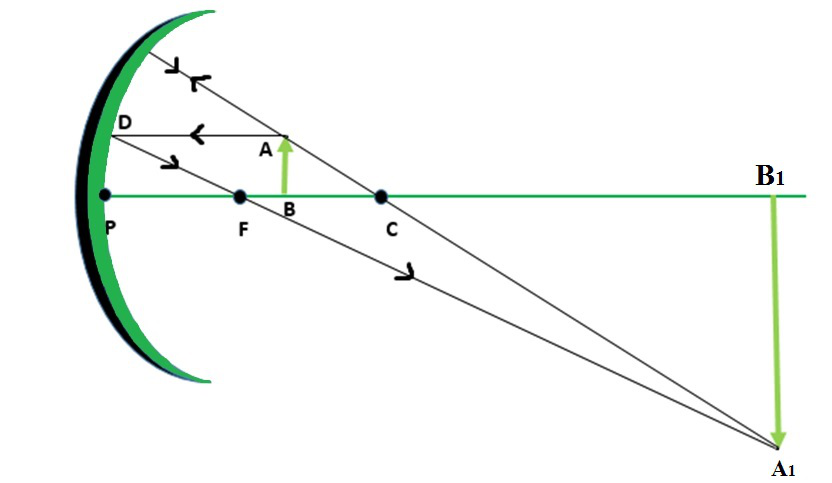• Object at Principal Focus (F):

When the object is placed at the principal focus (F) of a concave mirror, a highly enlarged image of object is formed at infinity.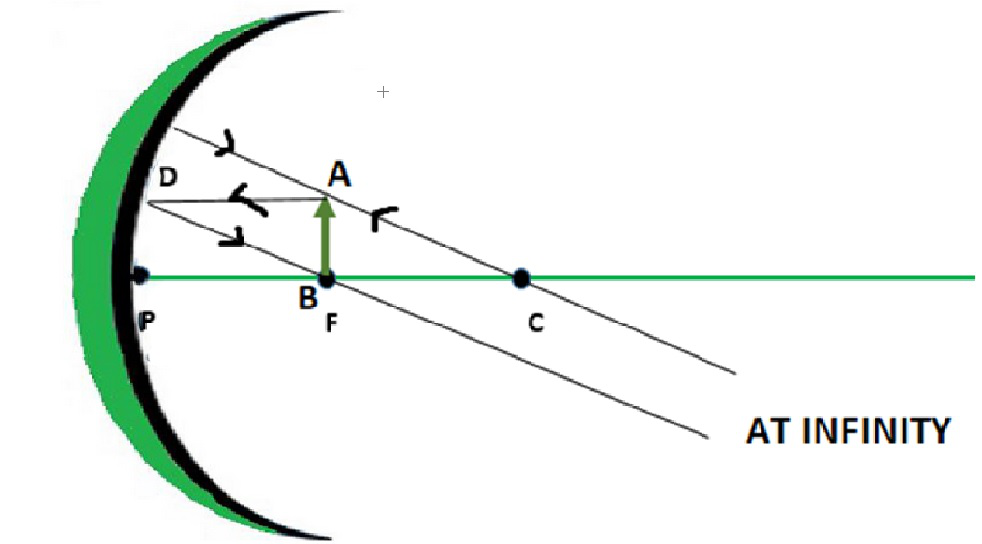Properties of image: Highly enlarged image, its nature is real and inverted

• Object between Principal Focus (F) and Pole (P):

When the object is placed anywhere between the principal focus and the pole of a concave mirror, we get an enlarged, virtual and erect image formed behind the mirror.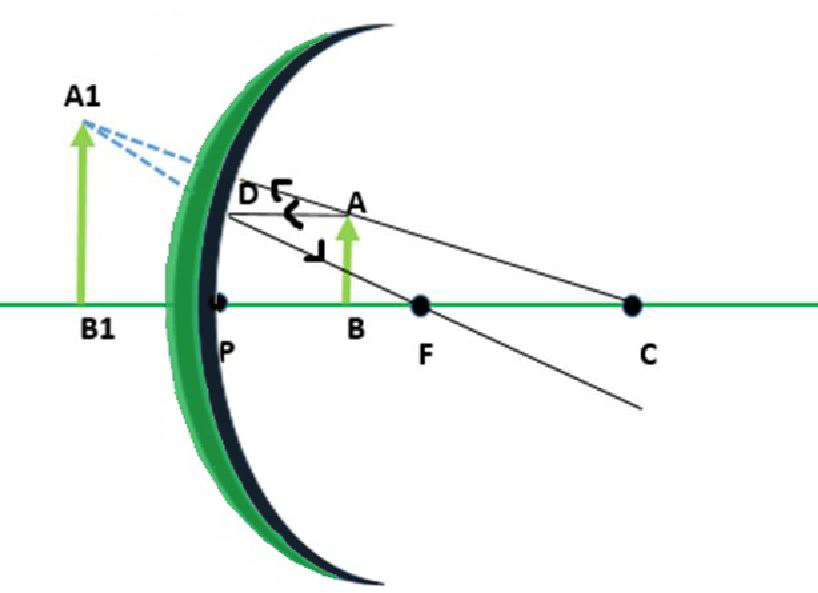Object between F and P

Properties of image: Enlarged, Virtual and erect.

### Convex Mirror

If the cut part of the hollow sphere is painted from inside, then its outer surface becomes the reflecting surface. This mirror is known as a convex mirror. A spherical mirror having its reflecting surface curved outwards is known to be a convex mirror. The back of the mirror are shaded so that reflection only takes place from the outward bulged part. The surface of the spoon which is bulged outwards can be assumed to be a convex mirror. It is also known as a diverging mirror as the light after reflecting through its surface diverges to many directions but appears to meet at some points where the virtual, erect image of diminished size is formed.

Properties Of Convex Mirror:

• A convex or diverging mirror is also known as a diverging mirror as this mirror diverges light when they strike on its reflecting surface.
• Virtual, erect, and diminished images are always formed or observed with convex mirrors, irrespective of the distance between the object and the mirror.

Application of Convex Mirror:

• Convex mirrors used inside buildings: Large offices, stores, and hospitals use a convex mirror to let people see around the corner so that they can avoid running into each other and prevent any collision.
• Convex mirror used in vehicles: Convex mirrors are commonly used as rear-view mirrors in case of automobiles and vehicles because it can diverge light beams and make virtual images.
• Uses of the convex mirror in a magnifying glass: These mirrors are mostly used for making magnifying glasses. In industries, to construct a magnifying glass, two convex mirrors are placed back to back.
• Convex mirrors used for security purposes: Diverging mirrors are also used for security purposes in many places. They are places near ATMs so that bank customers can check if someone is behind them.
• Convex mirrors are also used in various other places like as a street light reflectors as it can spread light over bigger areas.

### Image Formation By Concave Mirror And Their Ray Diagrams

Two possibilities of the position of the object are possible in the case of a convex mirror, which is when the object at infinity and the object between infinity and the pole of a convex mirror.

• Object at infinity

Whenever the object is kept at infinity, we observe that a point-sized image is formed at principal focus behind the convex mirror.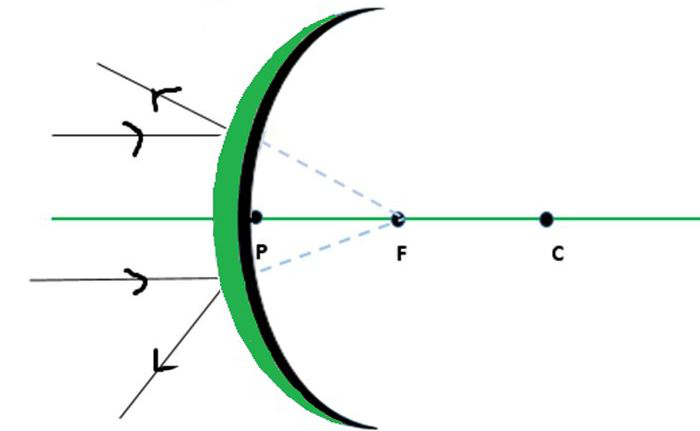Object at infinity

Properties of image: Image formed is highly diminished in size, virtual and erect

• Object is kept between infinity and pole

Whenever the object is kept anywhere between the infinity and the pole of a convex mirror, then we get a diminished, virtual and erect image formed between the pole and focus behind the mirror.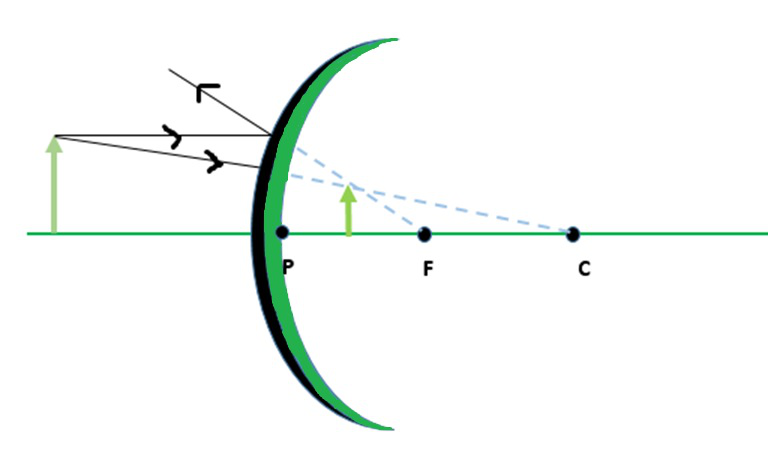Fig: Object between infinity and P

Properties of image: Image formed is diminished in size as well as virtual and erect.

### Mirror Formula

To do the sums related to the spherical mirrors, the formula used is known as the mirror formula. It is used to calculate the focal length, image distance, object distance, and also the magnification or any other thing required. We usually put the formula first and then put the signs so as to do the sums to minimize any error which can be generated. The sign conventions which are to be followed while using the mirror formula are fixed so from the above-given diagram we can easily put the signs according to the requirement to get the required result.

The formula is given below,Where u = object distance, v = image distance, f = focal length of mirror.

### Sign Conventions for Spherical Mirrors

Usually, if the object is located on the left side of the principal axis from the mirror then the object distance is taken negative. While if it is located on the right side it is taken to be positive. The sign of focal length depends on the type of mirror we are using, as for the concave mirror it is negative and for the convex mirror on the other hand is positive always. It is to be mentioned again that we have to follow the sign conventions strictly to get the correct answer. Heights which are above the principal axis are positive and below are negative.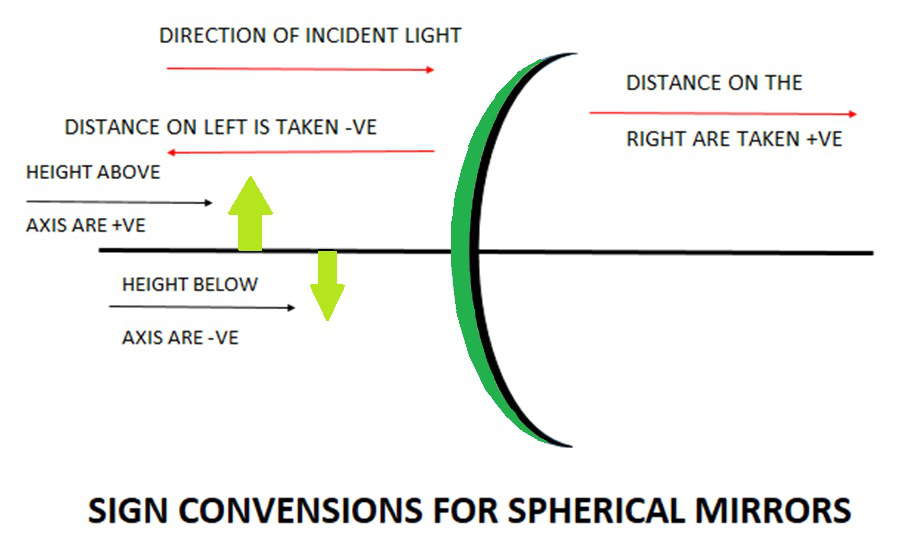### Sample Problems

Question 1: What is the image distance in case of concave mirror if the object distance is 4 cm? It is given that the focal length of the mirror is 2 cm.

Solution:

As we know from mirror formula,Where u= object distance= -4cm

v= image distance=?

f= focal length of mirror= -2cm

Putting values we getTherefore, v=-4 cm

Hence the object is located 4cm in front of the mirror.

Question 2: What is the image distance in case of concave mirror if the object distance is 32 cm? It is given that the focal length of the mirror is 16 cm. State the nature and the size of the image which is formed.

Solution:

As we know from mirror formula,Where u= object distance= -32cm

v= image distance=?

f= focal length of mirror= -16cm

Putting values we getv= -16 cm

Hence the object is located 8 cm in front of the mirror. And the image formed is real and inverted. As it is located at the centre of curvature hence the size of the image is also same as that of object.

Question 3: What is the image distance in case of convex mirror if the object distance is 12 cm? It is given that the focal length of the mirror is 12 cm.

Solution:

As we know from mirror formula,Where u= object distance= -12cm

v= image distance=?

f= focal length of mirror= 12cm

Putting values we getv=6 cm

Hence the image is located 6cm behind the mirror. The nature of the image is virtual and erect and it is between the focus and the pole behind the mirror. The size of the image is found to be diminished.

Question 4: What is the image distance in case of concave mirror if the object distance is 10 cm? It is given that the focal length of the mirror is 10 cm.

Solution:

As we know from mirror formula,Where u= object distance= -10cm

v= image distance=?

f= focal length of mirror= -10cm

Putting values we getv= infinity

Hence the image will be formed at infinity.

Question 5: What is the image distance in case of convex mirror if the object is at the focus of the mirror? It is given that the focal length of the mirror is 10 cm. What can u say about the nature of the image formed and also the size?

Solution:

As we know from mirror formula,Where u= object distance= -10cm

v= image distance=?

f= focal length of mirror= +10cm

Putting values we getv= 5 cm

Hence the image is located 5 cm behind the mirror. The image formed is virtual and erect and size is diminished.

Question 6: What are the applications of convex mirror? State any two.

Solution:

Following are the two application of convex mirror:

1. As rear view mirrors in vehicles
2. As magnifying glass

Question 7:  What is the image distance in case of convex mirror if the object distance is 16 cm? It is given that the focal length of the mirror is 16 cm. State the nature of the image which is formed and also give the size of it.

Solution:

As we know from mirror formula,Where u= object distance= -16cm

v= image distance=?

f= focal length of mirror= 16cm

Putting values we getv= 8 cm

Hence the image is located 8 cm behind the mirror. The nature of the image is virtual and erect and it is between the focus and the pole behind the mirror. The size of the image is found to be diminished.

My Personal Notes arrow_drop_up